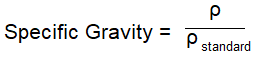﻿ Specific Gravity Calculator ﻿# Specific Gravity CalculatorWhat Composition is the Substance? Solid Liquid Gas Enter Density of the Substance, ρ:

This specific gravity calculator calculates the specific gravity of a substance.

The specific gravity is the ratio of the density of the substance to the density of some standard substance.

The standard substance is usually water (at 4°C) for liquids and solids, while for gases, it is usually air.

The density of water is 0.9998395 g/ml at 4.0° Celsius (39.2° Fahrenheit). Many places will approximate the density of water to 1 g/mL, but to be more precise, it actually is 0.9998395 g/ml.

The density of air is 1.225 kg/m3. This is usaually the density of the standard substance that is used for gases.

To use this calculator, a user chooses the composition of the substance s/he wants to find the specific gravity for, whether a solid, liquid, or gas and enters the density of the substance and clicks the 'Calculate' button, and the specific gravity will automatically be computed and shown below. The density can be entered in any units and the resultant answer will be dimensionless, because all the units to compute the computation cancel out. Specific gravity is a dimensionless resultant, because the 2 densities being compared against each other have the same units.

Specific gravity tells us whether the density of an object, if for a solid or liquid, is denser than water. The specific gravity tells us whether the density of an object, if it is a gas, is denser than air.

Thus, the specific gravity gives us good information when comparing the specific gravity of one object to standard densities of other objects, such as water and air.

Related Resources

﻿